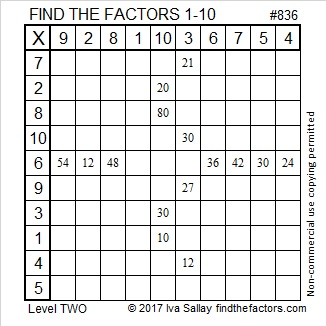# Finding Ways to Write 836 as the Sum of Consecutive Numbers

OEIS.org informs us that 836² = 698,896, a palindrome.Print the puzzles or type the solution on this excel file: 10-factors-835-842

836 can be written as the sum of 11 consecutive numbers and as the sum of 19 consecutive numbers because 11 and 19 are its odd factors (not including 1) that are less than 41. (Remember 861 is the 41st triangular number.) Notice 836’s factor pairs highlighted in red.

• 71 + 72 + 73 + 74 + 75 + 76 + 77 + 78 + 79 + 80 + 81 = 836; that’s 11 consecutive numbers
• 35 + 36 + 37 + 38 + 39 + 40 + 41 + 42 + 43 + 44 + 45 + 46 + 47 + 48 + 49 + 50 + 51 + 52 + 53 = 836; that’s 19 consecutive numbers

836 can be written as the sum of 8 consecutive numbers. Why? Because its factor that is the greatest power of 2 is 4, and because 1 is a factor of 836. Note that 2(4)(1) = 8.

• 101 + 102 + 103 + 104 + 105 + 106 + 107 + 108 = 836

836 can’t be written as the sum of 2(4)(11) = 88 consecutive numbers or 2(4)(19) = 152 consecutive numbers because neither 88 or 152 is less than 41.

• 836 is a composite number.
• Prime factorization: 836 = 2 × 2 × 5 × 41, which can be written 836 = 2² × 11 × 19
• The exponents in the prime factorization are 2, 1, and 1. Adding one to each and multiplying we get (2 + 1)(1 + 1)(1 + 1) = 3 × 2 × 2 = 12. Therefore 836 has exactly 12 factors.
• Factors of 836: 1, 2, 4, 11, 19, 22, 38, 44, 76, 209, 418, 836
• Factor pairs: 836 = 1 × 836, 2 × 418, 4 × 209, 11 × 76, 19 × 44, or 22 × 38
• Taking the factor pair with the largest square number factor, we get √836 = (√4)(√209) = 2√209 ≈ 28.91366This site uses Akismet to reduce spam. Learn how your comment data is processed.